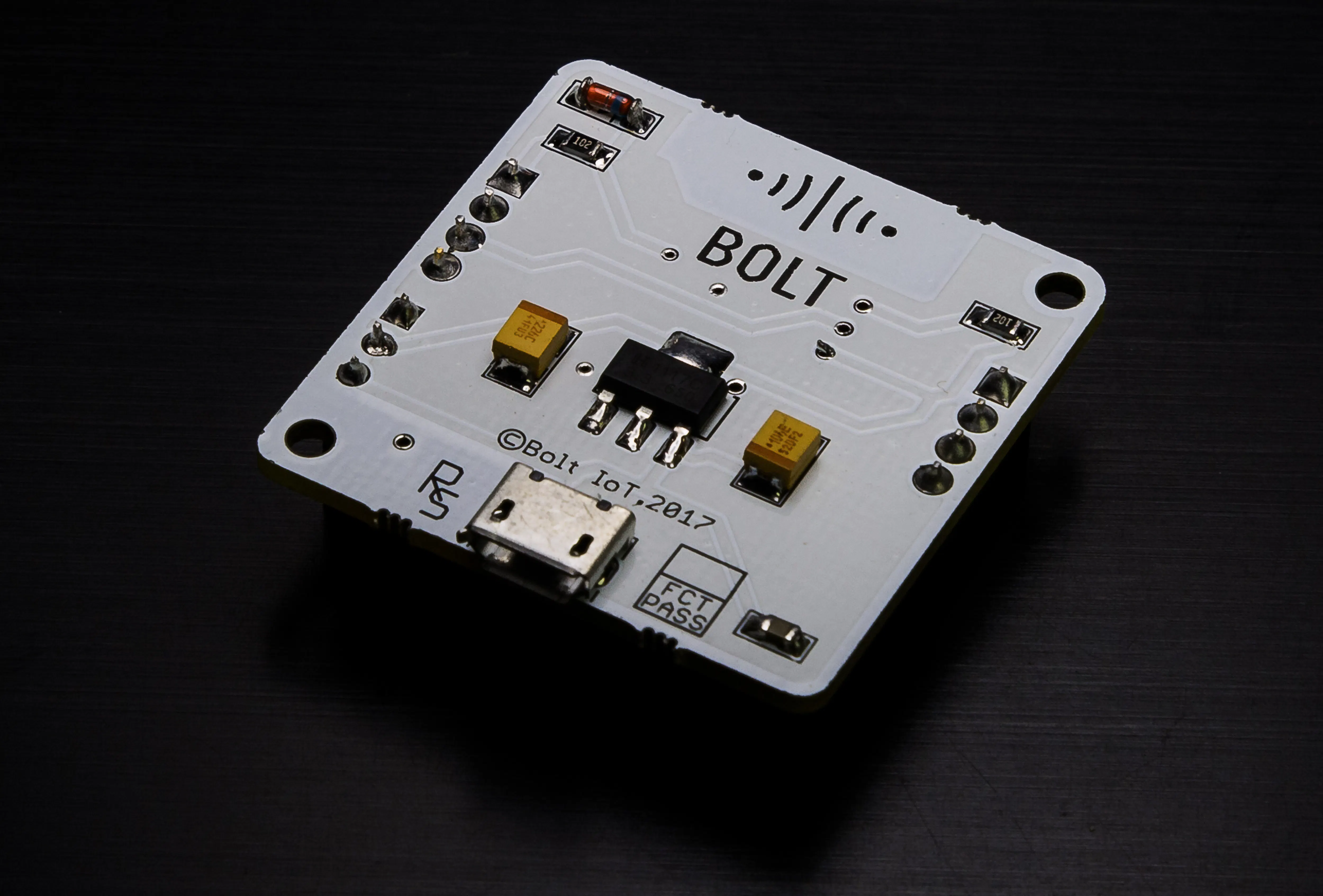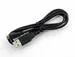Published

# Anomaly Detection with Light Intensity Monitoring Sensor

Anomaly detection using Z-score analysis with a light intensity monitoring sensor which sends alert messages on reaching threshold values.

IntermediateFull instructions provided5 hours594## Things used in this project

### Hardware componentsBolt IoT Bolt WiFi Module
×1USB-A to Micro-USB Cable
×1LDR, 5 Mohm
×1Resistor 10k ohm
×1

### Software apps and online servicesBolt IoT Bolt Cloud
 digitalocean droplet
 VmWare workstation
 virtual boxTwilio SMS Messaging API

Read more

## Code

### Anomaly_detection

Python
```import conf, json, time, math, statistics
from boltiot import Sms, Bolt
def compute_bounds(history_data,frame_size,factor):
if len(history_data)<frame_size :
return None

if len(history_data)>frame_size :
del history_data[0:len(history_data)-frame_size]
Mn=statistics.mean(history_data)
Variance=0
for data in history_data :
Variance += math.pow((data-Mn),2)
Zn = factor * math.sqrt(Variance / frame_size)
High_bound = history_data[frame_size-1]+Zn
Low_bound = history_data[frame_size-1]-Zn
return [High_bound,Low_bound]

mybolt = Bolt(conf.API_KEY, conf.DEVICE_ID)
sms = Sms(conf.SSID, conf.AUTH_TOKEN, conf.TO_NUMBER, conf.FROM_NUMBER)
history_data=[]

while True:
response = mybolt.analogRead('A0')
data = json.loads(response)
if data['success'] != 1:
print("There was an error while retriving the data.")
print("This is the error:"+data['value'])
time.sleep(10)
continue

print ("This is the value "+data['value'])
sensor_value=0
try:
sensor_value = int(data['value'])
except e:
print("There was an error while parsing the response: ",e)
continue

bound = compute_bounds(history_data,conf.FRAME_SIZE,conf.MUL_FACTOR)
if not bound:
required_data_count=conf.FRAME_SIZE-len(history_data)
print("Not enough data to compute Z-score. Need ",required_data_count," more data points")
history_data.append(int(data['value']))
time.sleep(10)
continue

try:
if sensor_value > bound :
print ("The light level increased suddenly. Sending an SMS.")
response = sms.send_sms("Decrese the brightness of the lights")
print("This is the response ",response)
elif sensor_value < bound:
print ("The light level decreased suddenly. Sending an SMS.")
response = sms.send_sms("Increse the brightness off the lights")
print("This is the response ",response)
history_data.append(sensor_value);
except Exception as e:
print ("Error",e)
time.sleep(10)
```

### twillo_messenger

Python
```SSID = 'You can find SSID in your Twilio Dashboard'
AUTH_TOKEN = 'You can find  on your Twilio Dashboard'
FROM_NUMBER = 'This is the no. generated by Twilio. You can find this on your Twilio Dashboard'
TO_NUMBER = 'This is your number. Make sure you are adding +91 in beginning'
API_KEY = 'This is your Bolt Cloud account API key'
DEVICE_ID = 'This is the ID of your Bolt device'
FRAME_SIZE = 10
MUL_FACTOR = 6
```

### Prediction

JavaScript
```setChartLibrary('google-chart');
setChartTitle('Polynomial Regression');
setChartType('predictionGraph');
setAxisName('time_stamp','temp');
mul(0.0977);
plotChart('time_stamp','temp');
```

## Credits

### Rahul

1 project • 0 followers